# hashMap 能抗多深入追问 总结篇

## 基础知识

hashMap 的进阶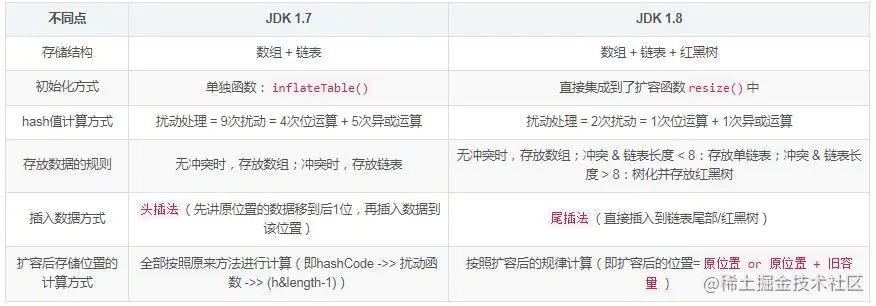在JDK1.6，JDK1.7中，HashMap采用位桶+链表实现，即使用链表处理冲突，  同一hash值的链表都存储在一个链表里。但是当位于一个桶中的元素较多，即hash值相等的元素较多时，通过key值依次查找的效率较低。而JDK1.8中，HashMap采用位桶+链表+红黑树实现，当链表长度超过阈值（8）时，将链表转换为红黑树，这样大大减少了查找时间。

# 简单说下HashMap的实现原理

HashMap的流程图是：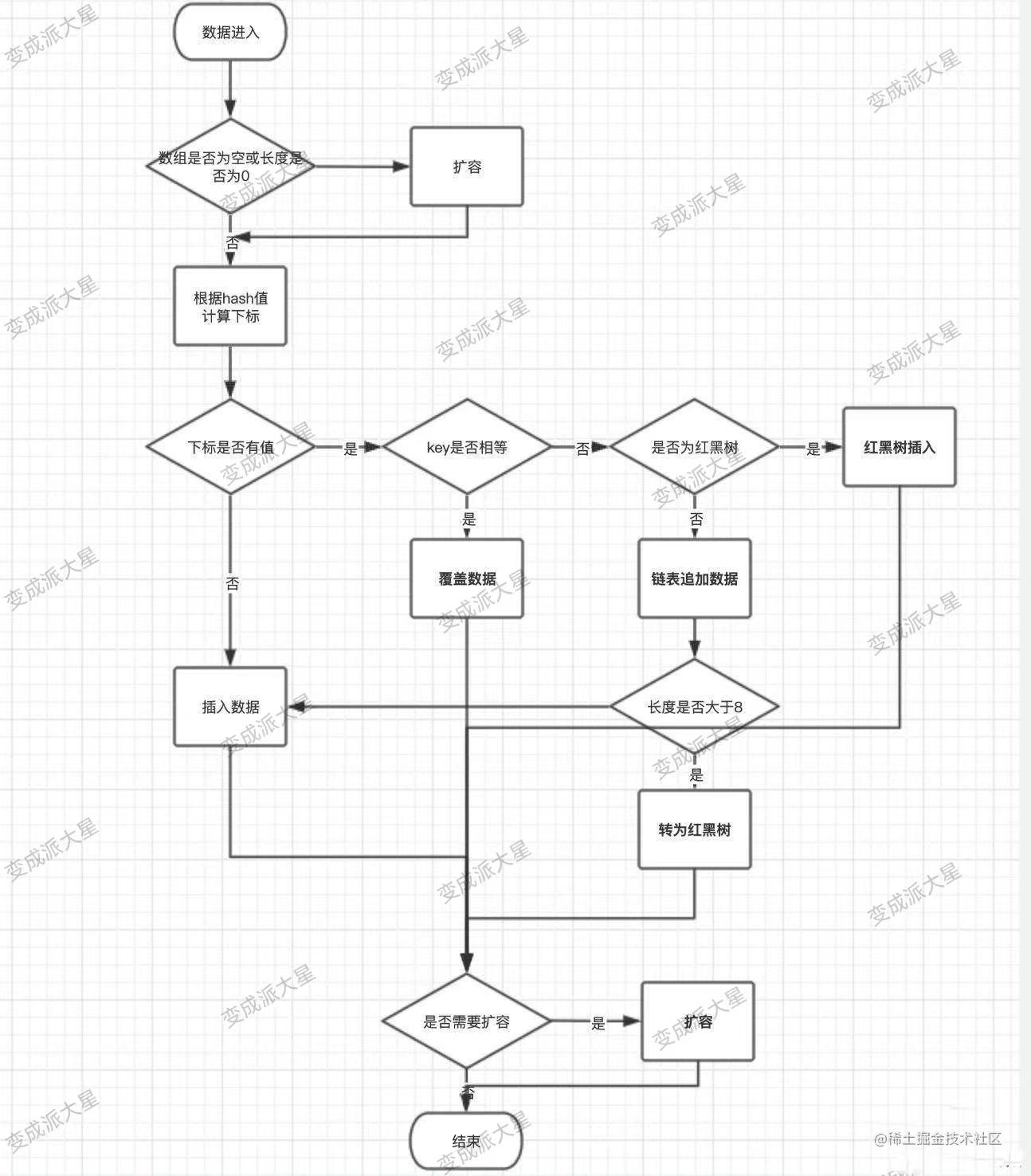# 一，JDK1.8中的涉及到的数据结构

## 1，位桶数组

`transient Node<k,v>[] table;//存储（位桶）的数组</k,v>`

## 2，数组元素Node实现了Entry接口

``` //Node是单向链表，它实现了Map.Entry接口 static class Node implements Map.Entry { final int hash; final K key; V value; Node next;

``````//构造函数Hash值 键 值 下一个节点
Node(int hash, K key, V value, Node<k,v> next) {
this.hash = hash;
this.key = key;
this.value = value;
this.next = next;
}

public final K getKey()        { return key; }
public final V getValue()      { return value; }
public final String toString() { return key + = + value; }

public final int hashCode() {
return Objects.hashCode(key) ^ Objects.hashCode(value);
}

public final V setValue(V newValue) {
V oldValue = value;
value = newValue;
return oldValue;
}

//判断两个node是否相等,若key和value都相等，返回true。可以与自身比较为true
public final boolean equals(Object o) {
if (o == this)
return true;
if (o instanceof Map.Entry) {
Map.Entry<!--?,?--> e = (Map.Entry<!--?,?-->)o;
if (Objects.equals(key, e.getKey()) &&
Objects.equals(value, e.getValue()))
return true;
}
return false;
}
``````

```

## 3，红黑树

``` //红黑树 static final class TreeNode extends LinkedHashMap.Entry { TreeNode parent; // 父节点 TreeNode left; //左子树 TreeNode right;//右子树 TreeNode prev; // needed to unlink next upon deletion boolean red; //颜色属性 TreeNode(int hash, K key, V val, Node next) { super(hash, key, val, next); }

``````//返回当前节点的根节点
final TreeNode<k,v> root() {
for (TreeNode<k,v> r = this, p;;) {
if ((p = r.parent) == null)
return r;
r = p;
}
}
``````

```

# 二，源码中的数据域

HashMap本来是以空间换时间，所以填充比没必要太大。但是填充比太小又会导致空间浪费。如果关注内存，填充比可以稍大，如果主要关注查找性能，填充比可以稍小。

``` public class HashMap extends AbstractMap implements Map, Cloneable, Serializable { private static final long serialVersionUID = 362498820763181265L;

``````// aka 16
static final int DEFAULT_INITIAL_CAPACITY = 1 << 4;

//最大容量
static final int MAXIMUM_CAPACITY = 1 << 30;

//填充比
static final float DEFAULT_LOAD_FACTOR = 0.75f;

static final int TREEIFY_THRESHOLD = 8;
static final int UNTREEIFY_THRESHOLD = 6;
static final int MIN_TREEIFY_CAPACITY = 64;

//存储元素的数组
transient Node<k,v>[] table;
transient Set<map.entry<k,v>> entrySet;

//存放元素的个数
transient int size;

//被修改的次数fast-fail机制
transient int modCount;

//临界值 当实际大小(容量*填充比)超过临界值时，会进行扩容
int threshold;

//填充比（......后面略）
``````

```

# 三，HashMap的构造函数

HashMap的构造方法有4种，主要涉及到的参数有，指定初始容量，指定填充比和用来初始化的Map

``` //构造函数1 public HashMap(int initialCapacity, float loadFactor) {

``````//指定的初始容量非负
if (initialCapacity < 0)
throw new IllegalArgumentException(Illegal initial capacity:  +
initialCapacity);

//如果指定的初始容量大于最大容量,置为最大容量
if (initialCapacity > MAXIMUM_CAPACITY)
initialCapacity = MAXIMUM_CAPACITY;

//填充比为正
throw new IllegalArgumentException(Illegal load factor:  +
this.threshold = tableSizeFor(initialCapacity);//新的扩容临界值
``````

}

//构造函数2 public HashMap(int initialCapacity) { this(initialCapacity, DEFAULT_LOAD_FACTOR); }

//构造函数3 public HashMap() { this.loadFactor = DEFAULT_LOAD_FACTOR; // all other fields defaulted }

//构造函数4用m的元素初始化散列映射 public HashMap(Map m) { this.loadFactor = DEFAULT_LOAD_FACTOR; putMapEntries(m, false); } ```

# 四，HashMap的存取机制

## 1，HashMap如何getValue值，

```public V get(Object key) { Node<K,V> e; return (e = getNode(hash(key), key)) == null ? null : e.value; } /** * Implements Map.get and related methods * * @param hash hash for key * @param key the key * @return the node, or null if none */ final Node<K,V> getNode(int hash, Object key) { Node<K,V>[] tab;//Entry对象数组 Node<K,V> first,e; //在tab数组中经过散列的第一个位置 int n; K k; /*找到插入的第一个Node，方法是hash值和n-1相与，tab[(n - 1) & hash]*/ //也就是说在一条链上的hash值相同的 if ((tab = table) != null && (n = tab.length) > 0 &&(first = tab[(n - 1) & hash]) != null) { /*检查第一个Node是不是要找的Node*/ if (first.hash == hash && // always check first node ((k = first.key) == key || (key != null && key.equals(k))))//判断条件是hash值要相同，key值要相同 return first; /*检查first后面的node*/ if ((e = first.next) != null) { if (first instanceof TreeNode) return ((TreeNode<K,V>)first).getTreeNode(hash, key); /*遍历后面的链表，找到key值和hash值都相同的Node*/ do { if (e.hash == hash && ((k = e.key) == key || (key != null && key.equals(k)))) return e; } while ((e = e.next) != null); } } return null; }```

get(key)方法时获取key的hash值，计算hash&(n-1)得到在链表数组中的位置first=tab[hash&(n-1)],先判断first的key是否与参数key相等，不等就遍历后面的链表找到相同的key值返回对应的Value值即可

## 2，HashMap如何put(key，value);

```public V put(K key, V value) { return putVal(hash(key), key, value, false, true); } /** * Implements Map.put and related methods * * @param hash hash for key * @param key the key * @param value the value to put * @param onlyIfAbsent if true, don't change existing value * @param evict if false, the table is in creation mode. * @return previous value, or null if none */ final V putVal(int hash, K key, V value, boolean onlyIfAbsent, boolean evict) { Node<K,V>[] tab; Node<K,V> p; int n, i; if ((tab = table) == null || (n = tab.length) == 0) n = (tab = resize()).length; /*如果table的在（n-1）&hash的值是空，就新建一个节点插入在该位置*/ if ((p = tab[i = (n - 1) & hash]) == null) tab[i] = newNode(hash, key, value, null); /*表示有冲突,开始处理冲突*/ else { Node<K,V> e; K k; /*检查第一个Node，p是不是要找的值*/ if (p.hash == hash &&((k = p.key) == key || (key != null && key.equals(k)))) e = p; else if (p instanceof TreeNode) e = ((TreeNode<K,V>)p).putTreeVal(this, tab, hash, key, value); else { for (int binCount = 0; ; ++binCount) { /*指针为空就挂在后面*/ if ((e = p.next) == null) { p.next = newNode(hash, key, value, null); //如果冲突的节点数已经达到8个，看是否需要改变冲突节点的存储结构，　　　　　　　　　　　　　 　　　　　　　　　　　　//treeifyBin首先判断当前hashMap的长度，如果不足64，只进行 //resize，扩容table，如果达到64，那么将冲突的存储结构为红黑树 if (binCount >= TREEIFY_THRESHOLD - 1) // -1 for 1st treeifyBin(tab, hash); break; } /*如果有相同的key值就结束遍历*/ if (e.hash == hash &&((k = e.key) == key || (key != null && key.equals(k)))) break; p = e; } } /*就是链表上有相同的key值*/ if (e != null) { // existing mapping for key，就是key的Value存在 V oldValue = e.value; if (!onlyIfAbsent || oldValue == null) e.value = value; afterNodeAccess(e); return oldValue;//返回存在的Value值 } } ++modCount; /*如果当前大小大于门限，门限原本是初始容量*0.75*/ if (++size > threshold) resize();//扩容两倍 afterNodeInsertion(evict); return null; }```

1. 判断键值对数组tab[]是否为空或为null，否则以默认大小resize()；
2. 根据键值key计算hash值得到插入的数组索引i，如果tab[i]==null，直接新建节点添加，否则转入3
3. 判断当前数组中处理hash冲突的方式为链表还是红黑树(check第一个节点类型即可),分别处理

# 五，HasMap的扩容机制resize();

``` /* * Initializes or doubles table size. If null, allocates in * accord with initial capacity target held in field threshold. * Otherwise, because we are using power-of-two expansion, the * elements from each bin must either stay at same index, or move * with a power of two offset in the new table. * * @return the table / final Node[] resize() { Node[] oldTab = table; int oldCap = (oldTab == null) ? 0 : oldTab.length; int oldThr = threshold; int newCap, newThr = 0;

``````/*如果旧表的长度不是空*/
if (oldCap > 0) {
if (oldCap >= MAXIMUM_CAPACITY) {
threshold = Integer.MAX_VALUE;
return oldTab;
}
/*把新表的长度设置为旧表长度的两倍，newCap=2*oldCap*/
else if ((newCap = oldCap << 1) < MAXIMUM_CAPACITY &&
oldCap >= DEFAULT_INITIAL_CAPACITY)
/*把新表的门限设置为旧表门限的两倍，newThr=oldThr*2*/
newThr = oldThr << 1; // double threshold
}
/*如果旧表的长度的是0，就是说第一次初始化表*/
else if (oldThr > 0) // initial capacity was placed in threshold
newCap = oldThr;
else {               // zero initial threshold signifies using defaults
newCap = DEFAULT_INITIAL_CAPACITY;
}

if (newThr == 0) {
float ft = (float)newCap * loadFactor;//新表长度乘以加载因子
newThr = (newCap < MAXIMUM_CAPACITY && ft < (float)MAXIMUM_CAPACITY ?
(int)ft : Integer.MAX_VALUE);
}
threshold = newThr;
@SuppressWarnings({"rawtypes","unchecked"})
/*下面开始构造新表，初始化表中的数据*/
Node<K,V>[] newTab = (Node<K,V>[])new Node[newCap];
table = newTab;//把新表赋值给table
if (oldTab != null) {//原表不是空要把原表中数据移动到新表中
/*遍历原来的旧表*/
for (int j = 0; j < oldCap; ++j) {
Node<K,V> e;
if ((e = oldTab[j]) != null) {
oldTab[j] = null;
if (e.next == null)//说明这个node没有链表直接放在新表的e.hash & (newCap - 1)位置
newTab[e.hash & (newCap - 1)] = e;
else if (e instanceof TreeNode)
((TreeNode<K,V>)e).split(this, newTab, j, oldCap);
/*如果e后边有链表,到这里表示e后面带着个单链表，需要遍历单链表，将每个结点重*/
else { // preserve order保证顺序
新计算在新表的位置，并进行搬运
Node<K,V> loHead = null, loTail = null;
Node<K,V> hiHead = null, hiTail = null;
Node<K,V> next;

do {
next = e.next;//记录下一个结点
//新表是旧表的两倍容量，实例上就把单链表拆分为两队，
``````

//e.hash&oldCap为偶数一队，e.hash&oldCap为奇数一对 if ((e.hash & oldCap) == 0) { if (loTail == null) loHead = e; else loTail.next = e; loTail = e; } else { if (hiTail == null) hiHead = e; else hiTail.next = e; hiTail = e; } } while ((e = next) != null);

``````                    if (loTail != null) {//lo队不为null，放在新表原位置
loTail.next = null;
}
if (hiTail != null) {//hi队不为null，放在新表j+oldCap位置
hiTail.next = null;
}
}
}
}
}
return newTab;
}
``````

```

# 六，JDK1.8使用红黑树的改进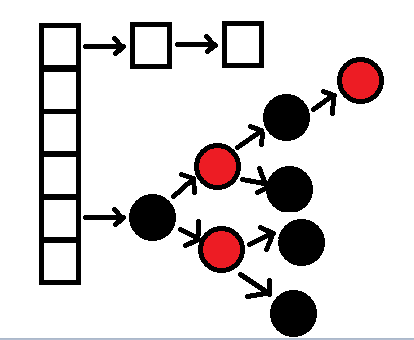JDK1.8HashMap的红黑树是这样解决的

## 常问面试题

### HashMap的长度为什么要是2的n次方

HashMap为了存取高效，要尽量较少碰撞，就是要尽量把数据分配均匀，每个链表长度大致相同，这个实现就在把数据存到哪个链表中的算法；\ 这个算法实际就是取模，`hash%length`，计算机中直接求余效率不如位移运算，源码中做了优化`hash&(length-1)`，\ `hash%length==hash&(length-1)`的前提是length是2的n次方；\ 为什么这样能均匀分布减少碰撞呢？2的n次方实际就是1后面n个0，2的n次方-1  实际就是n个1；\ 例如长度为9时候，`3&(9-1)=0`  `2&(9-1)=0` ，都在0上，碰撞了；\ 例如长度为8时候，`3&(8-1)=3`  `2&(8-1)=2` ，不同位置上，不碰撞；

```0000 0011     3 & 0000 1000      8 = 0000 0000      0```

```0000 0010     2 & 0000 1000    8 = 0000 0000      0```

```0000 0011     3 & 0000 0111     7 = 0000 0011       3```

```0000 0010      2 & 0000 0111      7 = 0000 0010      2```

``` / * * 直接【求余】和【按位】运算的差别验证 / public static void main(String[] args) { long currentTimeMillis = System.currentTimeMillis(); int a=0; int times = 1000010000;

``````for (long i = 0; i < times; i++) {
a=9999%1024;
}

long currentTimeMillis2 = System.currentTimeMillis();

int b=0;
for (long i = 0; i < times; i++) {
b=9999&(1024-1);
}

long currentTimeMillis3 = System.currentTimeMillis();

System.out.println(a+","+b);
System.out.println("%: "+(currentTimeMillis2-currentTimeMillis));
System.out.println("&: "+(currentTimeMillis3-currentTimeMillis2));
``````

} ```

```783,783 %: 359 &: 93```

### hashMap 中怎么判断是否存在相同Key

java中hashmap(key,value)的key和value都可以是null

```T t = map.get(key); if (t == null) { //key不存在，或者存的值是null } else { //key存在 }```

``` if (map.containskey(key)) { //key 存在 } else { //不存在 }

T t = map.get(key); if (t == null) { //存的值是null } ```

``` if (map.containskey(key)) { //key 存在 } else { //不存在 }

T t = map.get(key); if (t == null) { //存的值是null } ```

### hashMap resize 方法执行了什么

``` final Node[] resize() { Node[] oldTab = table; //当前所有元素所在的数组，称为老的元素数组 int oldCap = (oldTab == null) ? 0 : oldTab.length; //老的元素数组长度 int oldThr = threshold; // 老的扩容阀值设置 int newCap, newThr = 0; // 新数组的容量，新数组的扩容阀值都初始化为0 if (oldCap > 0) { // 如果老数组长度大于0，说明已经存在元素 // PS1 if (oldCap >= MAXIMUM_CAPACITY) { // 如果数组元素个数大于等于限定的最大容量（2的30次方） // 扩容阀值设置为int最大值（2的31次方 -1 ），因为oldCap再乘2就溢出了。 threshold = Integer.MAX_VALUE;
return oldTab; // 返回老的元素数组 }

``````   /*
* 如果数组元素个数在正常范围内，那么新的数组容量为老的数组容量的2倍（左移1位相当于乘以2）
* 如果扩容之后的新容量小于最大容量  并且  老的数组容量大于等于默认初始化容量（16），那么新数组的扩容阀值设置为老阀值的2倍。（老的数组容量大于16意味着：要么构造函数指定了一个大于16的初始化容量值，要么已经经历过了至少一次扩容）
*/
else if ((newCap = oldCap << 1) < MAXIMUM_CAPACITY &&
oldCap >= DEFAULT_INITIAL_CAPACITY)
newThr = oldThr << 1; // double threshold
}

// PS2
// 运行到这个else if  说明老数组没有任何元素
// 如果老数组的扩容阀值大于0，那么设置新数组的容量为该阀值
// 这一步也就意味着构造该map的时候，指定了初始化容量。
else if (oldThr > 0) // initial capacity was placed in threshold
newCap = oldThr;
else {               // zero initial threshold signifies using defaults
// 能运行到这里的话，说明是调用无参构造函数创建的该map，并且第一次添加元素
newCap = DEFAULT_INITIAL_CAPACITY;  // 设置新数组容量 为 16
newThr = (int)(DEFAULT_LOAD_FACTOR * DEFAULT_INITIAL_CAPACITY); // 设置新数组扩容阀值为 16*0.75 = 12。0.75为负载因子（当元素个数达到容量了4分之3，那么扩容）
}

// 如果扩容阀值为0 （PS2的情况）
if (newThr == 0) {
float ft = (float)newCap * loadFactor;
newThr = (newCap < MAXIMUM_CAPACITY && ft < (float)MAXIMUM_CAPACITY ?
(int)ft : Integer.MAX_VALUE);  // 参见：PS2
}
threshold = newThr; // 设置map的扩容阀值为 新的阀值
@SuppressWarnings({"rawtypes","unchecked"})
// 创建新的数组（对于第一次添加元素，那么这个数组就是第一个数组；对于存在oldTab的时候，那么这个数组就是要需要扩容到的新数组）
Node<K,V>[] newTab = (Node<K,V>[])new Node[newCap];
table = newTab; // 将该map的table属性指向到该新数组
if (oldTab != null) {   // 如果老数组不为空，说明是扩容操作，那么涉及到元素的转移操作
for (int j = 0; j < oldCap; ++j) { // 遍历老数组
Node<K,V> e;
if ((e = oldTab[j]) != null) { // 如果当前位置元素不为空，那么需要转移该元素到新数组
oldTab[j] = null; // 释放掉老数组对于要转移走的元素的引用（主要为了使得数组可被回收）
if (e.next == null) // 如果元素没有有下一个节点，说明该元素不存在hash冲突
// PS3
// 把元素存储到新的数组中，存储到数组的哪个位置需要根据hash值和数组长度来进行取模
// 【hash值  %   数组长度】   =    【  hash值   & （数组长度-1）】
//  这种与运算求模的方式要求  数组长度必须是2的N次方，但是可以通过构造函数随意指定初始化容量呀，如果指定了17,15这种，岂不是出问题了就？没关系，最终会通过tableSizeFor方法将用户指定的转化为大于其并且最相近的2的N次方。 15 -> 16、17-> 32
newTab[e.hash & (newCap - 1)] = e;

// 如果该元素有下一个节点，那么说明该位置上存在一个链表了（hash相同的多个元素以链表的方式存储到了老数组的这个位置上了）
// 例如：数组长度为16，那么hash值为1（1%16=1）的和hash值为17（17%16=1）的两个元素都是会存储在数组的第2个位置上（对应数组下标为1），当数组扩容为32（1%32=1）时，hash值为1的还应该存储在新数组的第二个位置上，但是hash值为17（17%32=17）的就应该存储在新数组的第18个位置上了。
// 所以，数组扩容后，所有元素都需要重新计算在新数组中的位置。

else if (e instanceof TreeNode)  // 如果该节点为TreeNode类型
((TreeNode<K,V>)e).split(this, newTab, j, oldCap);  // 此处单独展开讨论
else { // preserve order
Node<K,V> loHead = null, loTail = null;  // 按命名来翻译的话，应该叫低位首尾节点
Node<K,V> hiHead = null, hiTail = null;  // 按命名来翻译的话，应该叫高位首尾节点
// 以上的低位指的是新数组的 0  到 oldCap-1 、高位指定的是oldCap 到 newCap - 1
Node<K,V> next;
// 遍历链表
do {
next = e.next;
// 这一步判断好狠，拿元素的hash值  和  老数组的长度  做与运算
// PS3里曾说到，数组的长度一定是2的N次方（例如16），如果hash值和该长度做与运算，那么该hash值可参与计算的有效二进制位就是和长度二进制对等的后几位，如果结果为0，说明hash值中参与计算的对等的二进制位的最高位一定为0.
//因为数组长度的二进制有效最高位是1（例如16对应的二进制是10000），只有*..0**** 和 10000 进行与运算结果才为00000（*..表示不确定的多个二进制位）。又因为定位下标时的取模运算是以hash值和长度减1进行与运算，所以下标 = (*..0**** & 1111) 也= (*..0**** & 11111) 。1111是15的二进制、11111是16*2-1 也就是31的二级制（2倍扩容）。
// 所以该hash值再和新数组的长度取摸的话mod值也不会放生变化，也就是说该元素的在新数组的位置和在老数组的位置是相同的，所以该元素可以放置在低位链表中。
if ((e.hash & oldCap) == 0) {
// PS4
if (loTail == null) // 如果没有尾，说明链表为空
else
loTail.next = e; // 如果有尾，那么链表不为空，把该元素挂到链表的最后。
loTail = e; // 把尾节点设置为当前元素
}

// 如果与运算结果不为0，说明hash值大于老数组长度（例如hash值为17）
// 此时该元素应该放置到新数组的高位位置上
// 例：老数组长度16，那么新数组长度为32，hash为17的应该放置在数组的第17个位置上，也就是下标为16，那么下标为16已经属于高位了，低位是[0-15]，高位是[16-31]
else {  // 以下逻辑同PS4
if (hiTail == null)
else
hiTail.next = e;
hiTail = e;
}
} while ((e = next) != null);
if (loTail != null) { // 低位的元素组成的链表还是放置在原来的位置
loTail.next = null;
}
if (hiTail != null) {  // 高位的元素组成的链表放置的位置只是在原有位置上偏移了老数组的长度个位置。
hiTail.next = null;
newTab[j + oldCap] = hiHead; // 例：hash为 17 在老数组放置在0下标，在新数组放置在16下标；    hash为 18 在老数组放置在1下标，在新数组放置在17下标；
}
}
}
}
}
return newTab; // 返回新数组
``````

} ```

### 如何实现HashMap的有序？

`LinkedHashMap内部维护了一个单链表`，有头尾节点，`同时LinkedHashMap节点Entry内部除了继承HashMap的Node属性`，还有before 和 after用于标识前置节点和后置节点。可以实现按插入的顺序或访问顺序排序。

/* * The tail (youngest) of the doubly linked list. / transient LinkedHashMap.Entry tail; //将加入的p节点添加到链表末尾 private void linkNodeLast(LinkedHashMap.Entry p) { LinkedHashMap.Entry last = tail; tail = p; if (last == null) head = p; else { p.before = last; last.after = p; } } //LinkedHashMap的节点类 static class Entry extends HashMap.Node { Entry before, after; Entry(int hash, K key, V value, Node next) { super(hash, key, value, next); } } ```

```1:变成派大星 2:国庆快乐 3:掘金 4:跑步```

### HashMap是线程安全的吗？

• JDK1.7：会产生死循环、数据丢失、数据覆盖的问题；
• JDK1.8：中会有数据覆盖的问题。

#### 再问：你是如何解决这个线程不安全问题的？

• HashTable：是直接在操作方法上加synchronized关键字，锁住整个数组，粒度比较大；
• SynchronizedMap：是使用Collections集合工具的内部类，通过传入Map封装出一个SynchronizedMap对象，内部定义了一个对象锁，方法内通过对象锁实现；
• ConcurrentHashMap：使用分段锁（CAS + synchronized相结合），降低了锁粒度，大大提高并发度

#### 再问：什么情况下 使用 ConcurrentHashMap

`线程不安全的HashMap`

`效率低下的HashTable`

HashTable容器使用synchronized来保证线程安全，但在线程竞争激烈的情况下HashTable的效率非常低下（类似于数据库中的串行化隔离级别）。因为当一个线程访问HashTable的同步方法，其他线程也访问HashTable的同步方法时，会进入阻塞或轮询状态。如线程1使用put进行元素添加，线程2不但不能使用put方法添加元素，也不能使用get方法来获取元素，读写操作均需要获取锁，竞争越激烈效率越低。

`ConcurrentHashMap的分段锁技术可有效提升并发访问率`

HashTable容器在竞争激烈的并发环境下表现出效率低下的原因是所有访问HashTable的线程都必须竞争同一把锁，假如容器里有多把锁，每一把锁用于锁容器其中一部分数据，那么当多线程访问容器里不同数据段的数据时，线程间就不会存在严重锁竞争，从而可以有效提高并发访问效率，这就是ConcurrentHashMap所使用的分段锁技术。首先将数据分成一段一段地存储（一堆Segment），然后给每一段数据配一把锁，当一个线程占用锁访问其中一个段数据的时候，其他段的数据也能被其他线程访问。

• 默认数组大小为16
• 扩容因子为0.75，扩容后数组大小翻倍
• 当存储的node总数量 >= 数组长度*扩容因子时，会进行扩容（数组中的元素、链表元素、红黑树元素都是内部类Node的实例或子类实例，这里的node总数量是指所有put进map的node数量）
• 当链表长度>=8且数组长度<64时会进行扩容
• 当数组下是链表时，在扩容的时候会从链表的尾部开始rehash
• 当链表长度>=8且数组长度>=64时链表会变成红黑树
• 树节点减少直至为空时会将对应的数组下标置空，下次存储操作再定位在这个下标t时会按照链表存储
• 扩容时树节点数量<=6时会变成链表
• 当一个事 物 操作发现map正在扩容时，会帮助扩容
• map正在扩容时获取（get等类似操作）操作还没进行扩容的下标会从原来的table获取，扩容完毕的下标会从新的table中获取

### HashMap的死循环

#### 实现

HashMap的put方法实现：

`1、判断key是否已经存在`

```

public V put(K key, V value) { if (key == null) return putForNullKey(value); int hash = hash(key); int i = indexFor(hash, table.length); // 如果key已经存在，则替换value，并返回旧值 for (Entry e = table[i]; e != null; e = e.next) { Object k; if (e.hash == hash && ((k = e.key) == key || key.equals(k))) { V oldValue = e.value; e.value = value; e.recordAccess(this); return oldValue; } }

``````modCount++;
// key不存在，则插入新的元素
return null;
``````

} ```

`2、检查容量是否达到阈值threshold`

```

void addEntry(int hash, K key, V value, int bucketIndex) { if ((size >= threshold) && (null != table[bucketIndex])) { resize(2 * table.length); hash = (null != key) ? hash(key) : 0; bucketIndex = indexFor(hash, table.length); }

``````createEntry(hash, key, value, bucketIndex);
``````

} ```

`3、扩容实现`

```

void resize(int newCapacity) { Entry[] oldTable = table; int oldCapacity = oldTable.length; ...

``````Entry[] newTable = new Entry[newCapacity];
...
transfer(newTable, rehash);
table = newTable;
threshold = (int)Math.min(newCapacity * loadFactor, MAXIMUM_CAPACITY + 1);
``````

} ```

```

void transfer(Entry[] newTable, boolean rehash) { int newCapacity = newTable.length; for (Entry e : table) { while(null != e) { Entry next = e.next; if (rehash) { e.hash = null == e.key ? 0 : hash(e.key); } int i = indexFor(e.hash, newCapacity); e.next = newTable[i]; newTable[i] = e; e = next; } } } ```

#### 案例分析

```

void transfer(Entry[] newTable, boolean rehash) { int newCapacity = newTable.length; for (Entry e : table) { while(null != e) { Entry next = e.next; if (rehash) { e.hash = null == e.key ? 0 : hash(e.key); } int i = indexFor(e.hash, newCapacity); e.next = newTable[i]; newTable[i] = e; e = next; } } } ```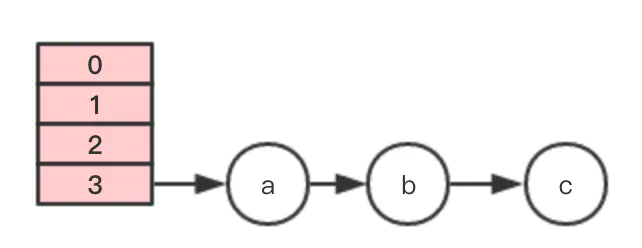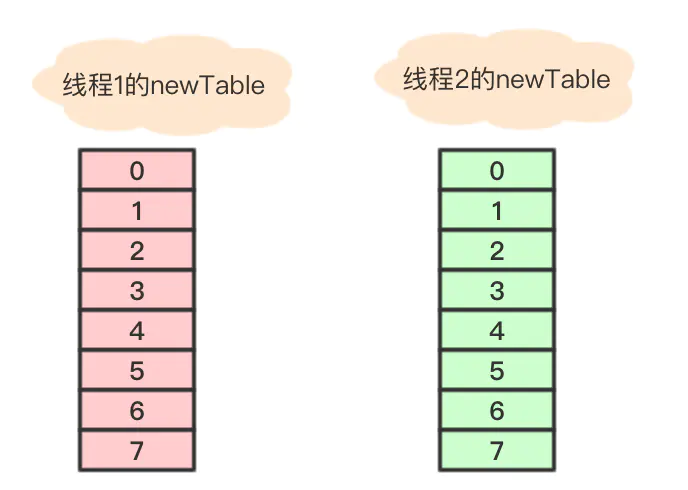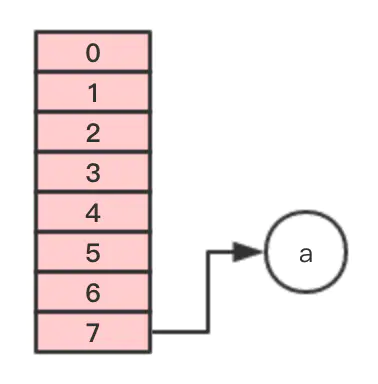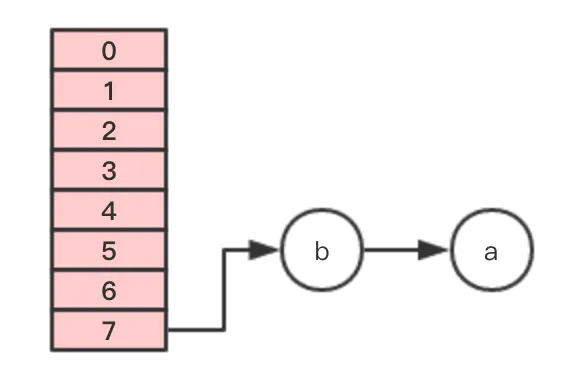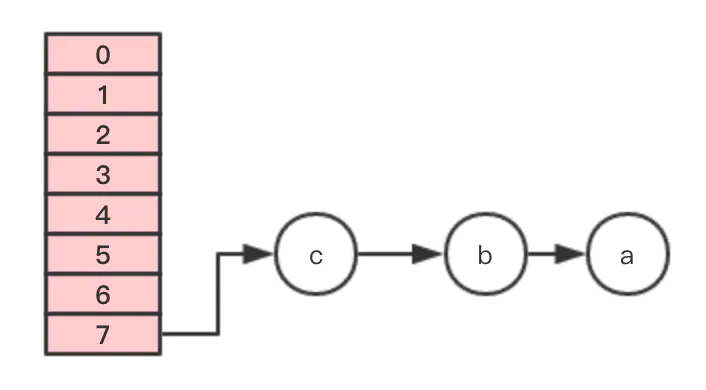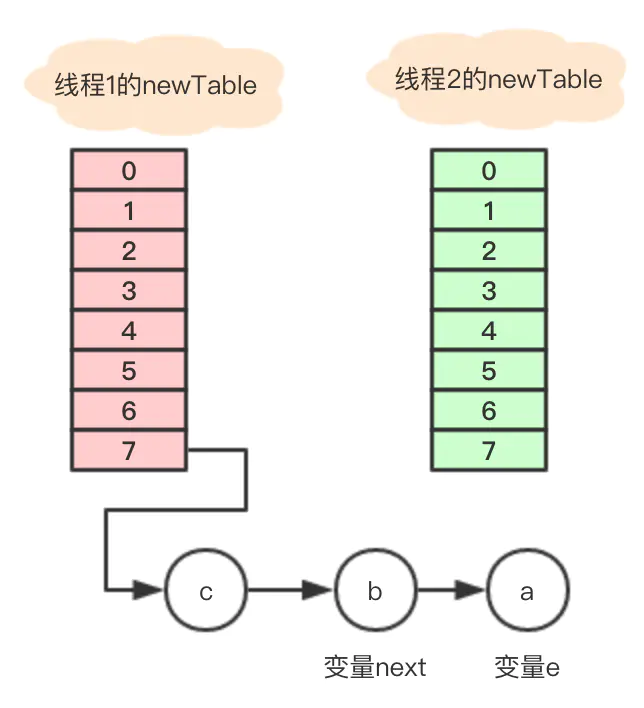```

Entry next = e.next; int i = indexFor(e.hash, newCapacity); e.next = newTable[i]; newTable[i] = e; e = next; ```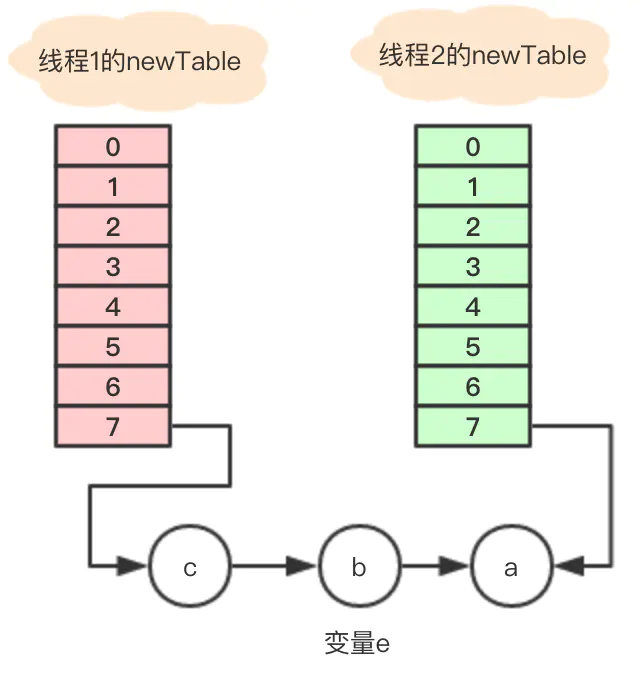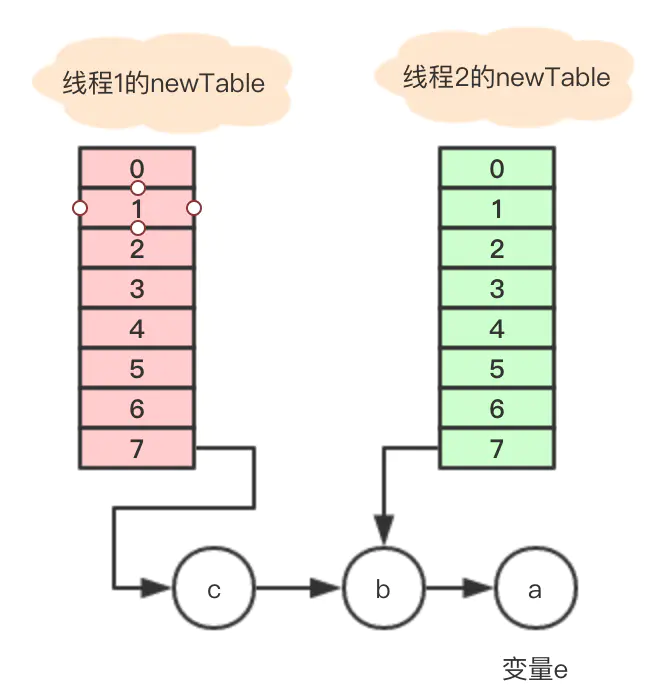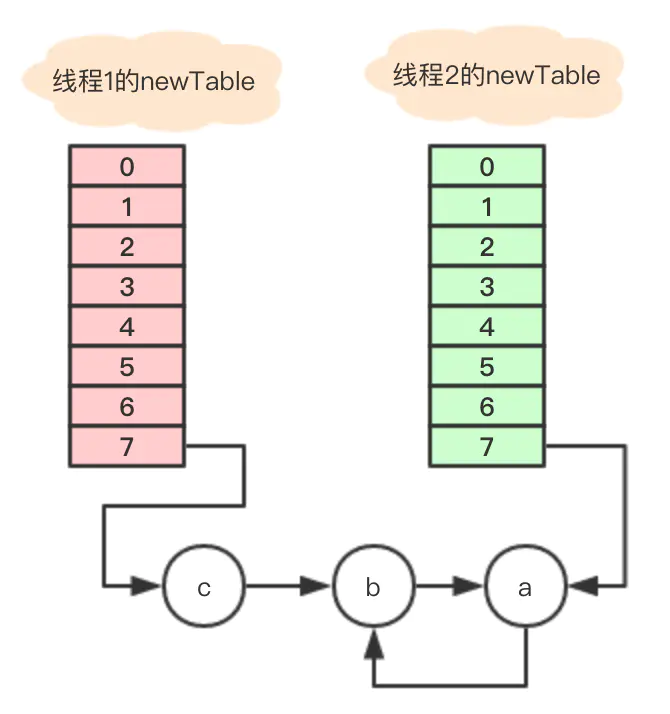「其他文章」